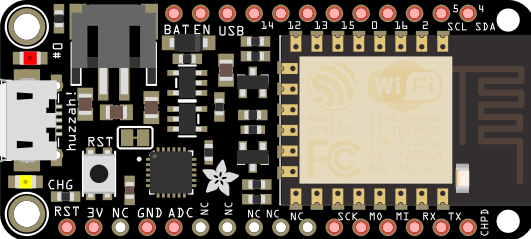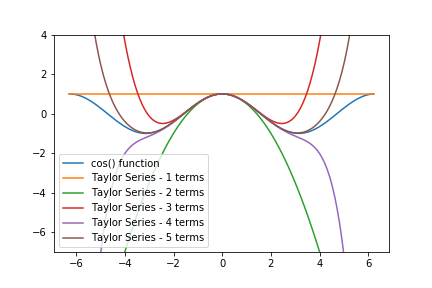## How to upload .py-files onto an ESP8266 running MicroPythonThis is an update to the sixth part of a multi-part series on Micropython. I had trouble in class today uploading a .py-file to an ESP8266 microcontroller running MicroPython using a command line tool called ampy. There was a trick getting ampy to work on with the ESP8266 microcontroller that …

## Taylor Series in PythonIn this post, we will review how to create a Taylor Series with Python and for loops. Then we will refactor the Taylor Series into functions and compare the output of our Taylor Series functions to functions from Python's Standard Library.

A Taylor Series is an infinite series of mathematical …

## Hear my story about moving from MATLAB to Python on TalkPython

I was a guest on the TalkPython podcast with Michael Kennedy! We talked about teaching Python at a Community College and how I moved a college engineering class from MATLAB to Python using JupyterHub.

## Plotting a Gaussian normal curve with Python and MatplotlibIn the previous post, we calculated the area under the standard normal curve using Python and the erf() function from the math module in Python's Standard Library. In this post, we will construct a plot that illustrates the standard normal curve and the area we calculated. To build the Gaussian …

## Calculate the Probability Under a Normal CurveCalculating the probability under a normal curve is useful for engineers. This type of calculation can be helpful to predict the likely hood of a part coming off an assembly line being within a given specification. The probability can be calculated when the statistical properties of all the parts that …